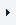LOGINREGISTER (FREE TRIAL)myTOMLAB
 OptimizationLinear ProgrammingQuadratic ProgrammingUnconstrained OptimizationConstrained Optimization, Nonlinear ProgrammingLinear Least SquaresConstrained Nonlinear Least SquaresBox-bounded Global OptimizationGlobal Optimization, costly functionsMixed-Integer ProgrammingMixed-Integer Quadratic ProgrammingMixed-Integer Nonlinear ProgrammingSemi-definite Programming

# Unconstrained Optimization (convex or non-convex)

 TOMLAB /KNITRO TOMLAB /SNOPT - SNOPT

The TOMLAB Base Module routine ucSolve includes several of the most popular search step methods for unconstrained optimization. Problems with simple bounds on the unknown variables are considered as unconstrained, and solvable by ucSolve. Any nonlinear programming solver used to solve constrained optimization problems is also usable for unconstrained problems.

If the problem is very large (hundreds of thousands of variables), barrier (interior point) methods most often performs better. A good choice if the problem is convex is PDCO in the TOMLAB Base Module. For a general non-convex objective TOMLAB /KNITRO is the preferred choice.

If the problem has less than 100 000 variables, a solver like SNOPT in TOMLAB /SNOPT might also be applicable. An efficient solver for small-scale, dense problems is NPSOL in TOMLAB /NPSOL

Solver reference:

 TOMLAB /NPSOL - NPSOL TOMLAB Base Module - PDCO TOMLAB Base Module - ucSolve